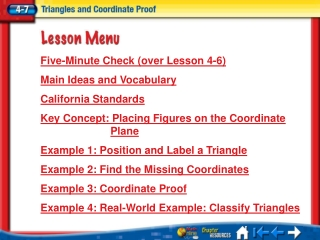Download PresentationTélécharger la présentation- - - - - - - - - - - - - - - - - - - - - - - - - - - E N D - - - - - - - - - - - - - - - - - - - - - - - - - - -
Presentation Transcript

1. Five-Minute Check (over Lesson 4-6) Main Ideas and Vocabulary California Standards Key Concept: Placing Figures on the CoordinatePlane Example 1: Position and Label a Triangle Example 2: Find the Missing Coordinates Example 3: Coordinate Proof Example 4: Real-World Example: Classify Triangles Lesson 7 Menu

2. Position and label triangles for use in coordinate proofs. • Write coordinate proofs. • coordinate proof Lesson 7 MI/Vocab

3. Standard 17.0Students prove theorems by using coordinate geometry, including the midpoint of a line segment, the distance formula,and various forms of equations of lines and circles. (Key) Lesson 7 CA

4. Lesson 7 KC1

5. Position and label right triangleXYZ with leg d units long on the coordinate plane. Position and Label a Triangle Use the origin as vertex X of the triangle. Place the base of the triangle along the positive x-axis. Position the triangle in the first quadrant. Since Z is on the x-axis, its y-coordinate is 0. Its x-coordinate is d because the base is d units long. Lesson 7 Ex1

6. Position and Label a Triangle Since triangle XYZ is a right triangle the x-coordinate of Y is 0. We cannot determine the y-coordinate so call it b. Answer: Animation: Placing Figures on the Coordinate Plane for Coordinate Proofs Lesson 7 Ex1

7. Which picture on the following slide would be the best way to position and label equilateral triangle ABC with side w units long on the coordinate plane? • A • B • C • D Lesson 7 CYP1

8. A.B. C.D. • A • B • C • D Lesson 7 CYP1

9. The y-coordinate for S is the distance from R to S. Since ΔQRS is an isosceles right triangle, Find the Missing Coordinates Name the missing coordinates of isosceles right triangle QRS. Q is on the origin, so its coordinates are (0, 0). The x-coordinate of S is the same as the x-coordinate for R, (c, ?). The distance from Q to R is c units. The distance from R to S must be the same. So, the coordinates of S are (c, c). Answer:Q(0, 0); S(c, c) Lesson 7 Ex2

10. Name the missing coordinates of isosceles right ΔABC. • A • B • C • D A.A(d, 0); C(0, 0) B.A(0, f); C(0, 0) C.A(0, d); C(0, 0) D.A(0, 0); C(0, d) Lesson 7 CYP2

11. Given:ΔXYZ is isosceles. Coordinate Proof Write a coordinate proof to prove that the segment that joins the vertex angle of an isosceles triangle to the midpoint of its base is perpendicular to the base. The first step is to position and label an isosceles triangle on the coordinate plane. Place the base of the isosceles triangle along the x-axis. Draw a line segment from the vertex of the triangle to its base. Label the origin and label the coordinates, using multiples of 2 since the Midpoint Formula takes half the sum of the coordinates. Prove: Lesson 7 Ex3

12. Proof:By the Midpoint Formula, the coordinates of W, the midpoint of , is The slope of or undefined. The slope of is therefore, . Coordinate Proof Lesson 7 Ex3

13. Finish the following coordinate proof to prove that the segment drawn from the right angle to the midpoint of the hypotenuse of an isosceles right triangle is perpendicular to the hypotenuse. Lesson 7 CYP3

14. Proof:The coordinates of the midpoint D are The slope of is or 1. The slope of or –1, therefore because ____. ? • A • B • C • D A. their slopes are opposite. B. the sum of their slopes is zero. C. the product of their slopes is –1. D. the difference of their slopes is 2. Lesson 7 CYP3

15. Classify Triangles DRAFTINGWrite a coordinate proof to prove that the outside of this drafter’s tool is shaped like a right triangle. The length of one side is 10 inches and the length of another side is 5.75 inches. Lesson 7 Ex4

16. Proof:The slope of or undefined. The slope of or 0, therefore ΔDEF is a right triangle. The drafter’s tool is shaped like aright triangle. Classify Triangles Lesson 7 Ex4

17. FLAGS Write a coordinate proof to prove this flag is shaped like an isosceles triangle. The altitude is 16 inches and the base is 10 inches. Lesson 7 CYP4

18. What is the ordered pair for Point C? • A • B • C • D A. (10, 10) B. (10, 5) C. (16, 10) D. (16, 5) Lesson 7 CYP4

19. A.21 B.16 C. D.10 Next, determine the lengths of CA and CB. What are the lengths of both of these? • A • B • C • D Lesson 7 CYP4

20. End of Lesson 7